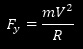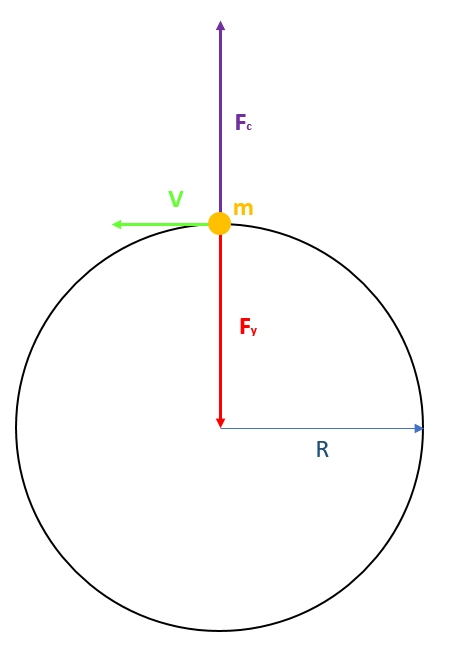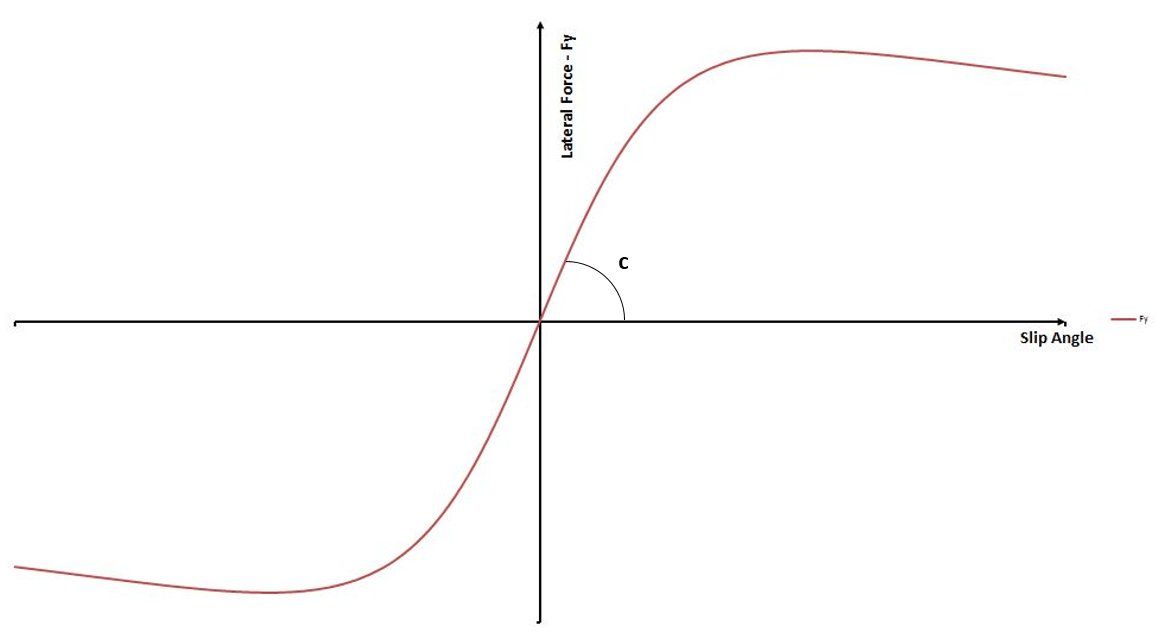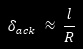[su_list icon=”icon: angle-double-right” icon_color=”#3498db”]

[/su_list]

We briefly went through an overview of the main phenomena involved with a car moving on a straight line. What we did is far from a complete analysis, but it should help the reader to get a feeling about some of the most important parameters to be considered when analyzing how a car accelerates in a straight line.

We will now apply a similar approach to analyze, again very briefly, what happens when a car negotiates a corner. Again, we will use a simplified approach, in order to make things a bit easier to understand and to focus on the most important points. Some of the topics we dealt with in our tyre article series will also be used here to explain how the car exchange forces with the road.

We will start considering a very simple body, with a mass “m”, that moves on a circular path, with a forward velocity “V”. To help visualize a similar situation, we could think about an object seating at the end of a rope, which we let rotate at a certain speed. If “R” is the radius of said circular path (the length of our rope), our body will experience a centripetal acceleration “Ay” that points toward the centre of the curved trajectory.The magnitude of Ay depends on the square of tangential velocity and on the radius R and is given by the following equation:As we know, any time that a body accelerates, there must be a force acting on it in the same direction of the acceleration and with a net magnitude given by body’s mass multiplied by acceleration magnitude. In our case, we will call this force “centripetal”, because it keeps the body at a constant distance to the center of the curved path as it moves. We denote it with “Fy” and it follows that:We also know that, in a certain point in time, our equilibrium will be ensured by the presence of a force that balances Fy and tries to pull the body out of its path. We will call this force “centrifugal” and its magnitude is given by:This is more or less what happens to a car, traveling on a circular path with radius R, at constant speed V. The force ensuring the car can keep moving on its path is exerted by the four tyres; each of them exchanges a (more or less) lateral force with the road, because of the mechanisms we have already described in some of our previous articles. Actually, the four forces we are dealing with all point to the same point, so they are not parallel to each other, but to keep our analysis simple, we assume for now that each tyre develops a lateral force perpendicular to car centre line.

Similarly to the above-mentioned mass, a car moving at a constant speed V, on a circular path with radius R, will be subjected to the action of a force Fy that the road applies on it. The magnitude of Fy will be given by the sum of the forces acting on each of the four contact patches.Let’s move a step further our simple-mass analysis and let’s imagine to have a simplified car, where the two wheels of the same axle are collapsed into a single one. In other words, we can assume the car having front and rear track widths equal to zero. To make this simplified model work for our analysis, we will also assume that the CG (Center of Mass) lies at ground height, so there is no load transfer.

The overall vehicle weight will stay, exactly as the mass distribution, defined in terms of CG longitudinal position (length of a and b in the following picture). This means, front and rear wheels of our vehicle model will have to carry the forces normally reacted respectively by the whole front and rear axle.

This vehicle model is often called a “bicycle model”, for obvious reasons. As a didactic tool, it is often used assuming front and rear wheels are the same. Their cornering performance is assumed to be comparable to the sum of that of the two tyres of the same axle.This model is normally used under the assumption that its front and rear tyres work in their linear range, producing cornering forces that are simply given by the product of cornering stiffness and slip angle. Cornering stiffness is the slope of the slip curve close to its intersection with the origin. In general, it depends on vertical load, because of the shape of the slip curve changes depending on the vertical forces acting on the tyre. In this model, anyway, the vertical load is always univocally defined, so it stays constant.

In the following picture, cornering stiffness is denoted as c and it is the tangent of the angle between the slip curve and the horizontal axis, close to the origin.With this approach, cornering force Fy of each axle can be calculated as:Where α is the slip angle. This assumption makes the description of what happens physically a bit easier, above all if we also assume that front and rear tyres are the same. Anyway, in general, what is important is the tyre cornering force Fy, no matter under which assumptions it is calculated.

In all what follows, we will always refer to steering angle as the angle at which the wheels are actually steered and we will never consider the steering wheel angle, which is the rotation angle of the steering wheel.

We will start analyzing how this simple vehicle negotiates a corner with radius R at a very low speed. In such a situation, the cornering forces that each of the two tyres have to exchange with the road are also very small (we have seen that the centripetal force depends on the square of the forward velocity) and, consequently, also the slip angles will be very small; we will assume that both front and rear slip angles are so small, that we can neglect them. This means, the cornering forces will be perpendicular to the wheel middle plane and it will also dictate the direction each wheel is actually pointing in.
δ will be our front wheel steering angle.This simple example is very useful to define an important parameter of car handling. As the car steers thanks to front wheel rotation, we can derive the steering angle needed to negotiate a corner under the given assumption as:δack is called Ackermann Angle. This name denotes the angle at which the front wheel needs to be steered to negotiate a corner of radius R, when the slip angles can be neglected, which means at a very low lateral acceleration (hence also at a very low speed). This metric will be very useful later, to define how the car handles. Ackermann’s name is also connected to steering systems, as we will discuss in another article.

Although the Ackermann angle is only a theoretical value, it helps us to understand why small cars (or, anyway, cars with a small wheelbase), can normally negotiate small radius corners with more ease. Its definition tells us that, the shorter the wheelbase, the smaller is the steering angle required to drive the car on a fixed radius circular path.

This simple model also helps us to define another important concept, which is the body slip angle β. This is the angle between the velocity vector V and the car middle line/plane. As we will see, this angle changes depending on the cornering condition and has a direct connection to both front and rear slip angles. As shown in the picture, the velocity vector V is always perpendicular to the corner radius R. The position of the of the turn-centre with respect to the vehicle changes depending on the direction of front and rear tyres forces. We will come to this later in more details.

Looking at the previous picture, we notice immediately that, with respect to car local coordinate system, the front tyre exchange a force with the road that has not only a lateral component, but also a longitudinal one that, in this simple case, depends only on the steering angle, because we assumed the slip angles being equal to zero. This means that the more we steer, the biggest is the force that our powertrain will have to overcome to move the car forward (although this is not shown in our picture). If corner radius is very big (and, hence, our steering angle is very small), the longitudinal component of the front tyre force would be so small that we could neglect it.

In this case, we could write the equilibrium equations for our simple vehicle as follows:The first equations tells us that the tyres have to exchange forces with the road whose sum is equal to the centrifugal force –mAy.

The second equation states that, for the car to negotiate a corner under the given assumptions, the net moment around the vertical axis must be equal to zero. This is the condition at the base of steady-state cornering definition, which is a situation very similar to what a car experiences when driving on the circular path of a given radius at constant forward velocity. In reality, a racecar finds itself very rarely in a similar situation; probably, the only moment where a car is close to this condition for a very short time is when it hits a corner apex, although it very much depends on the corner, on the driving style and on the driving line.

For a car to enter and exit a corner, actually, the net moment around the vertical axis (Yaw Moment, N) must be different from zero, as having a net, the non-zero yaw moment is exactly what makes the car changing its heading direction. To simplify our analysis, anyway, we will always assume that the net yaw moment is equal to zero when nothing else is specified.